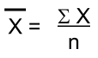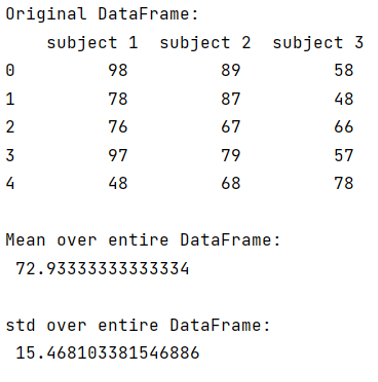# Pandas compute mean or std over entire dataframe

Given a pandas dataframe, we have to compute mean or std over entire dataframe.
Submitted by Pranit Sharma, on September 09, 2022

Pandas is a special tool that allows us to perform complex manipulations of data effectively and efficiently. Inside pandas, we mostly deal with a dataset in the form of DataFrame. DataFrames are 2-dimensional data structures in pandas. DataFrames consist of rows, columns, and data.

## How to compute mean or standard deviation (std) over entire DataFrame?

Mean is nothing but an average value of a series of a number. Mathematically, the mean can be calculated as:Here, is the mean, ∑x is the summation of all the values and n is the total number of values/elements.

Suppose we have a series of numbers from 1 to 10, then the average of this series will be:

```∑x = 1+2+3+4+5+6+7+8+9+10
∑x = 55
n = 10
x̄ = 55/10
x̄ = 5.5
```

But in pandas, we use pandas.DataFrame['col'].mean() directly to calculate the average value of a column.

But here, we need to calculate the mean over the entire DataFrame, for this purpose, we will access all the values of the DataFrame and then we will apply the nanmean() and nanstd() method which is a method of NumPy which calculates mean and standard deviation while ignoring nan values.

Let us understand with the help of an example,

## Python code to compute the mean or std over entire dataframe

```# Importing pandas package
import pandas as pd

# Importing numpy package
import numpy as np

# Creating a dictionary
d = {
'subject 1':[98,78,76,97,48],
'subject 2':[89,87,67,79,68],
'subject 3':[58,48,66,57,78]
}

# Creating DataFrame
df = pd.DataFrame(d)

# Display the DataFrame
print("Original DataFrame:\n",df,"\n")

# Calculating mean and std over
# entire DataFrame
res1 = np.nanmean(df.values)
res2 = np.nanstd(df.values)

# Display mean and std
print("Mean over entire DataFrame:\n",res1,"\n")
print("std over entire DataFrame:\n",res2,"\n")
```

Output:What's New (MCQs)

Top Interview Coding Problems/Challenges!

Languages: » C » C++ » C++ STL » Java » Data Structure » C#.Net » Android » Kotlin » SQL
Web Technologies: » PHP » Python » JavaScript » CSS » Ajax » Node.js » Web programming/HTML
Solved programs: » C » C++ » DS » Java » C#
Aptitude que. & ans.: » C » C++ » Java » DBMS
Interview que. & ans.: » C » Embedded C » Java » SEO » HR
CS Subjects: » CS Basics » O.S. » Networks » DBMS » Embedded Systems » Cloud Computing
» Machine learning » CS Organizations » Linux » DOS
More: » Articles » Puzzles » News/Updates

© https://www.includehelp.com some rights reserved.